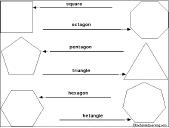EnchantedLearning.com is a user-supported site.
As a bonus, site members have access to a banner-ad-free version of the site, with print-friendly pages.

 AnswersMore on Shapes EnchantedLearning.comLabel the Regular Polygons More Label Me! printouts

Read the definitions, then label the regular polygons (many-sided geometric figures with equal-length sides and equal angles). The sum of the inner angles of a regular polygon is 180*(n-2) degrees, and each inner angle of a regular polygon is equal to [180*(n-2)]/n degrees, where n is the number of sides of the figure.This is a thumbnail of the polygons Label Me! diagram. The full-size printout is available only to site members.

equilateral triangle - a three-sided figure with equal length sides and with all three inner angles each equal to 60 degrees.

square - a four-sided figure in which each side is the same length and with all four interior angles are right angles (90 degrees).

regular pentagon - a five-sided figure with equal length sides and with inner angles each equal to 108 degrees.

regular hexagon - a six-sided figure with equal length sides and with inner angles each equal to 120 degrees.

regular heptagon - a seven-sided figure with equal length sides and with inner angles each equal to 128 4/7 degrees.

regular octagon - an eight-sided figure with equal length sides and with inner angles each equal to 135 degrees.

## Enchanted Learning Search

 Search the Enchanted Learning website for: